# Proof on how 0^0 = 1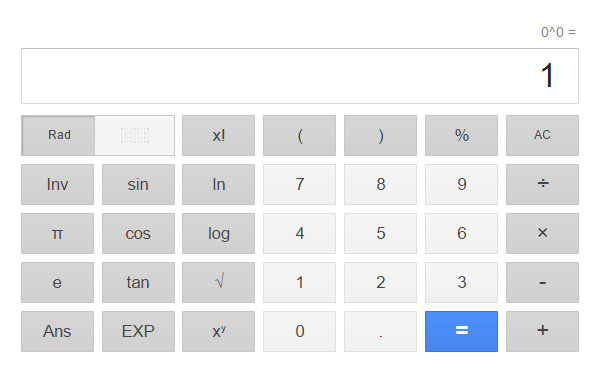According to many Calculus textbooks and calculus classes, 0^0 is an indeterminate form''. When evaluating a limit of the form 0^0, then you need to know that limits of that form are calledindeterminate forms'', and that you need to use a special technique such as L'Hopital's rule to evaluate them. Otherwise, 0^0 = 1 seems to be the most useful choice for 0^0. This convention allows us to extend definitions in different areas of mathematics that otherwise would require treating 0 as a special case. Notice that 0^0 is a discontinuity of the function x^y. More importantly, keep in mind that the value of a function and its limit need not be the same thing, and functions need not be continous, if that serves a purpose (see Dirac's Delta).

This means that depending on the context where 0^0 occurs, you might wish to substitute it with 1, indeterminate or undefined/nonexistent.

Some people feel that giving a value to a function with an essential discontinuity at a point, such as x^y at (0,0), is an inelegant patch and should not be done. Others point out correctly that in mathematics, usefulness and consistency are very important, and that under these parameters 0^0 = 1 is the natural choice.

The following is a list of reasons why 0^0 should be 1.

Rotando & Korn show that if f and g are real functions that vanish at the origin and are analytic at 0 (infinitely differentiable is not sufficient), then f(x)^(g(x)) approaches 1 as x approaches 0 from the right.

From Concrete Mathematics p.162 (R. Graham, D. Knuth, O. Patashnik):

Some textbooks leave the quantity 0^0 undefined, because the functions x^0 and 0^x have different limiting values when x decreases to 0. But this is a mistake. We must define x^0 = 1 for all x, if the binomial theorem is to be valid when x=0, y=0, and/or x=-y. The theorem is too important to be arbitrarily restricted! By contrast, the function 0^x is quite unimportant.

As a rule of thumb, one can say that 0^0 = 1, but 0.0^(0.0) is undefined, meaning that when approaching from a different direction there is no clearly predetermined value to assign to 0.0^(0.0); but Kahan has argued that 0.0^(0.0) should be 1, because if f(x), g(x) --> 0 as x approaches some limit, and f(x) and g(x) are analytic functions, then f(x)^g(x) --> 1.

The discussion on 0^0 is very old, Euler argues for 0^0 = 1 since a^0 = 1 for a != 0. The controversy raged throughout the nineteenth century, but was mainly conducted in the pages of the lesser journals: Grunert's Archiv and Schlomilch's Zeitschrift für Mathematik und Physik. Consensus has recently been built around setting the value of 0^0 = 1.

On a discussion of the use of the function 0^(0^x) by an Italian mathematician named Guglielmo Libri.

[T]he paper  did produce several ripples in mathematical waters when it originally appeared, because it stirred up a controversy about whether 0^0 is defined. Most mathematicians agreed that 0^0 = 1, but Cauchy [5, page 70] had listed 0^0 together with other expressions like 0/0 and oo - oo in a table of undefined forms. Libri's justification for the equation 0^0 = 1 was far from convincing, and a commentator who signed his name simply S'' rose to the attack . August Möbius  defended Libri, by presenting his former professor's reason for believing that 0^0 = 1 (basically a proof that lim_(x --> 0+) x^x = 1). Möbius also went further and presented a supposed proof that lim_(x --> 0+) f(x)^(g(x)) whenever lim_(x --> 0+) f(x) = lim_(x --> 0+) g(x) = 0. Of courseS'' then asked  whether Möbius knew about functions such as f(x) = e^(-1/x) and g(x) = x. (And paper  was quietly omitted from the historical record when the collected words of Möbius were ultimately published.) The debate stopped there, apparently with the conclusion that 0^0 should be undefined.

But no, no, ten thousand times no! Anybody who wants the binomial theorem (x + y)^n = sum_(k = 0)^n (n k) x^k y^(n - k) to hold for at least one nonnegative integer n must believe that 0^0 = 1, for we can plug in x = 0 and y = 1 to get 1 on the left and 0^0 on the right.

The number of mappings from the empty set to the empty set is 0^0. It has to be 1.

On the other hand, Cauchy had good reason to consider 0^0 as an undefined limiting form, in the sense that the limiting value of f(x)^(g(x)) is not known a priori when f(x) and g(x) approach 0 independently. In this much stronger sense, the value of 0^0 is less defined than, say, the value of 0+0. Both Cauchy and Libri were right, but Libri and his defenders did not understand why truth was on their side.

The alternating sum of binomial coefficients from the n-th row of Pascal's triangle is what you obtain by expanding (1-1)^n using the binomial theorem, i.e., 0^n. But the alternating sum of the entries of every row except the top row is 0, since 0^k=0 for all k greater than 1. But the top row of Pascal's triangle contains a single 1, so its alternating sum is 1, which supports the notion that (1-1)^0=0^0 if it were defined, should be 1.

The limit of x^x as x tends to zero (from the right) is 1. In other words, if we want the x^x function to be right continuous at 0, we should define it to be 1.

The expression m^n is the product of m with itself n times. Thus m^0, the "empty product", should be 1 (no matter what m is).

Another way to view the expression mn is as the number of ways to map an n-element set to an m-element set. For instance, there are 9 ways to map a 2-element set to a 3-element set. There are NO ways to map a 2-element set to the empty set (hence 0^2=0). However, there is exactly one way to map the empty set to itself: use the identity map! Hence 0^0=1.

Here's an aesthetic reason. A power series is often compactly expressed as SUMn=0 to INFINITY an (x-c)n.

We desire this expression to evaluate to a^0 when x=c, but the n=0 term in the above expression is problematic at x=c. This can be fixed by separating the a^0 term (not as nice) or by defining 0^0=1.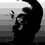Note by Lew Sterling Jr
6 years, 4 months ago

This discussion board is a place to discuss our Daily Challenges and the math and science related to those challenges. Explanations are more than just a solution — they should explain the steps and thinking strategies that you used to obtain the solution. Comments should further the discussion of math and science.

When posting on Brilliant:

• Use the emojis to react to an explanation, whether you're congratulating a job well done , or just really confused .
• Ask specific questions about the challenge or the steps in somebody's explanation. Well-posed questions can add a lot to the discussion, but posting "I don't understand!" doesn't help anyone.
• Try to contribute something new to the discussion, whether it is an extension, generalization or other idea related to the challenge.

MarkdownAppears as
*italics* or _italics_ italics
**bold** or __bold__ bold
- bulleted- list
• bulleted
• list
1. numbered2. list
1. numbered
2. list
Note: you must add a full line of space before and after lists for them to show up correctly
paragraph 1paragraph 2

paragraph 1

paragraph 2

[example link](https://brilliant.org)example link
> This is a quote
This is a quote
    # I indented these lines
# 4 spaces, and now they show
# up as a code block.

print "hello world"
# I indented these lines
# 4 spaces, and now they show
# up as a code block.

print "hello world"
MathAppears as
Remember to wrap math in $$ ... $$ or $ ... $ to ensure proper formatting.
2 \times 3 $2 \times 3$
2^{34} $2^{34}$
a_{i-1} $a_{i-1}$
\frac{2}{3} $\frac{2}{3}$
\sqrt{2} $\sqrt{2}$
\sum_{i=1}^3 $\sum_{i=1}^3$
\sin \theta $\sin \theta$
\boxed{123} $\boxed{123}$

Sort by:

@Llewellyn Sterling Obviously, the reason for saying n^0 = 1 is, n^2 = 1 x n x n n^1 = 1 x n = n^2 / n n^0 = (1xn)/n = 1 But in this case, n = 0. which implies n^0 = 1/0 which is undefined.

- 4 years, 10 months ago

I have checked a few sites just now and all are showing various answers.

Symbolab.com: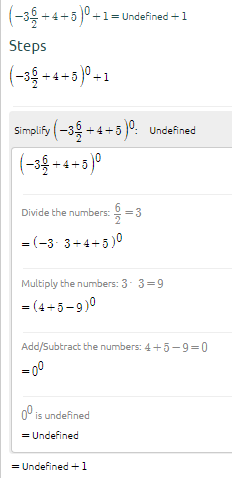Cymath.com: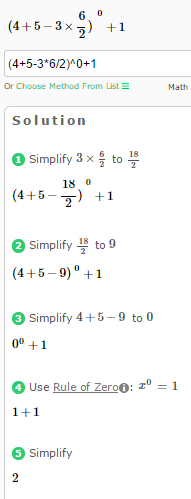WolframAlpha.com: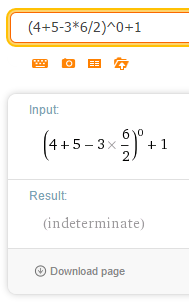- 6 years, 1 month ago

Just a question, a number raised to zero can be expressed as a^-1 * a^1 = a/a. Following the same logic 0^0 = 0/0. Now, is 0/0 = 1?

- 6 years, 4 months ago

Funny I was searching the internet and found proofs that $0^0=1$ then I came to this, thinking I can find more, but it looks exactly the same.

- 6 years, 4 months ago

0^0 Web 2.0: ERROR: INDETERMINATE 0^0 Google: 1 0^0 Scientific Cal. : ERROR

- 6 years, 4 months ago

I'm sorry...google says what for 0^0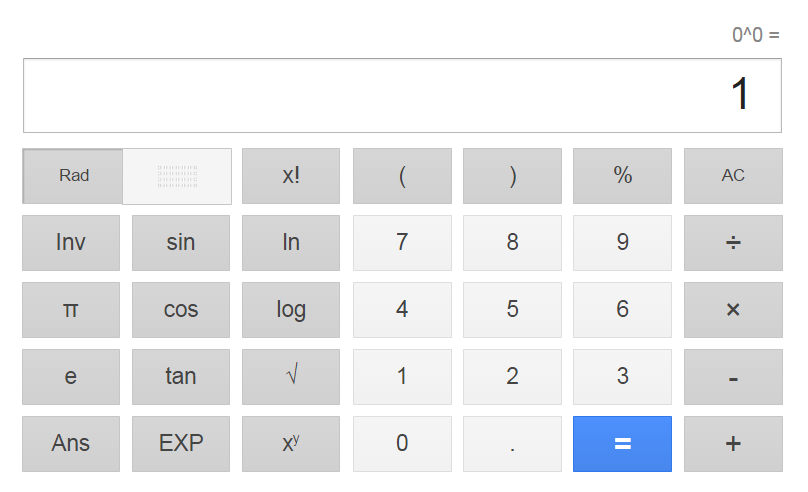- 6 years, 4 months ago

It is not necessary, can you find

$\lim_{x \to 0} x^{\dfrac{1}{logx}}$

It is of the form $0^0$ but the answer is not 1.

- 6 years, 4 months ago

So now what do we get as a final conclusion.... Is it 1 or undefined ???? Am still confused

- 6 years, 4 months ago

Limits:

From the left of 0, it is undefined.

From the right of 0, it is 10.

At exactly 0, it is 1.

I posted a graph of it just now. Look at my post.

- 6 years, 4 months ago

No am asking for 0 to the power of 0 .... Do we consider as 1 or 0

- 6 years, 4 months ago

1 is the multiplicative identity of real numbers.

If n > 0, then 0^n = 0, because you multiply 1 n times by 0.

n^0 is as simple as not multiplying 1 by n. Thus n^0 = 1 for any real number n.

- 6 years, 4 months ago

For any real number.

- 6 years, 1 month ago

In most calculus classes and textbooks, they say 0. However, its true answer would have to be 1.

- 6 years, 4 months ago

Ohk thanks

- 6 years, 4 months ago

Welcome. I am not really sure why they say in classes that it is zero.

Also, take a took at this that I just did from desmos.com/calculator.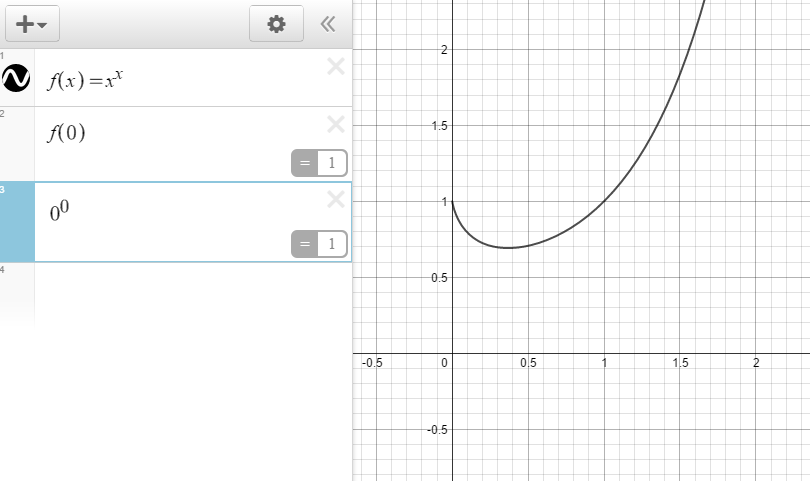- 6 years, 4 months ago

Funny; I got 10 as a final answer.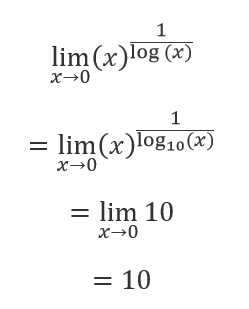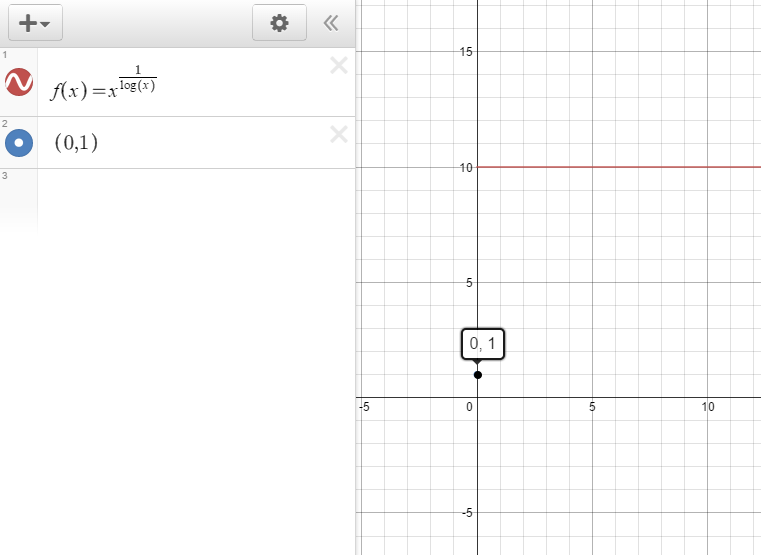- 6 years, 4 months ago